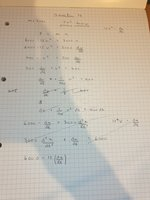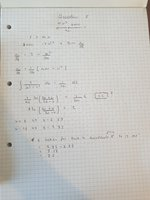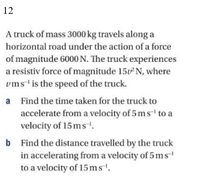# Non linear 1st order differential

#### Jomo

##### Elite Member
looks like a first order differential but it's v2 and i'm not sure how to do that.
What do you mean by this?
Can we see your work so we can help you? You would have received help by now if you followed the guidelines and shared your work with us.

#### H.Bisho18

##### New member
looks like a first order differential but it's v2 and i'm not sure how to do that.
What do you mean by this?
Can we see your work so we can help you? You would have received help by now if you followed the guidelines and shared your work with us.
sorry heres my working. I get tried making a first order differential which i couldn't solve and then tried a second order which i know is wrong#### MarkFL

##### Super Moderator
Staff member
I'd begin with the IVP:

$$\displaystyle \d{v}{t}=6000-15v^2=15(20^2-v^2)$$ where $$v(0)=5$$

The ODE is separable, and may be written in the following differential form:

$$\displaystyle \frac{1}{20^2-v^2}\,dv=15\,dt$$

What do we get upon applying a partial fraction decomposition on the LHS?

•Subhotosh Khan and Jomo

#### Jomo

##### Elite Member
sorry heres my working. I get tried making a first order differential which i couldn't solve and then tried a second order which i know is wrongView attachment 16003
When you divide the lhs of the equal sign by 200 you need to do the same to the rhs!

#### H.Bisho18

##### New member
I'd begin with the IVP:

$$\displaystyle \d{v}{t}=6000-15v^2=15(20^2-v^2)$$ where $$v(0)=5$$

The ODE is separable, and may be written in the following differential form:

$$\displaystyle \frac{1}{20^2-v^2}\,dv=15\,dt$$

What do we get upon applying a partial fraction decomposition on the LHS?
Ah yes i see now thank you!
However I did ignore +c when I intergrated as I couldn't see how you would determine it since the question doesnt say it starts at rest or anything.
Doing this I get the answer in the mark scheme but I was wondering if you know how you'd know c is 0 or was it just an oversight in the question?#### Subhotosh Khan

##### Super Moderator
Staff member
Ah yes i see now thank you!
However I did ignore +c when I intergrated as I couldn't see how you would determine it since the question doesnt say it starts at rest or anything.
Doing this I get the answer in the mark scheme but I was wondering if you know how you'd know c is 0 or was it just an oversight in the question?

View attachment 16014I do not see anywhere that "c" (I assume the c = constant of integration) was assumed to be zero - in response #5.

#### MarkFL

##### Super Moderator
Staff member
Ah yes i see now thank you!
However I did ignore +c when I intergrated as I couldn't see how you would determine it since the question doesnt say it starts at rest or anything.
Doing this I get the answer in the mark scheme but I was wondering if you know how you'd know c is 0 or was it just an oversight in the question?
Ah, yes, I failed to use Newton's 2nd law...the ODE should in fact be:

$$\displaystyle 3000\d{v}{t}=6000-15v^2$$

$$\displaystyle 200\d{v}{t}=20^2-v^2$$

I would continue as follows:

$$\displaystyle 5\left(\frac{1}{v+20}-\frac{1}{v-20}\right)\,dv=dt$$

Integrate, using the boundaries as limits and switch the dummy variables:

$$\displaystyle 5\int_5^v \frac{1}{y+20}-\frac{1}{y-20}\,dy=\int_0^t\,dz$$

$$\displaystyle 5\ln\left(\frac{3}{5}\left|\frac{v+20}{v-20}\right|\right)=t$$

And so, to answer the first question, we find:

$$\displaystyle t(15)=5\ln\left(\frac{21}{5}\right)\approx7.175$$

This agrees with your result, where you have taken $$v(0)=0$$. Under that model, the constant of integration would indeed be zero, but you don't want to, in practice, make that assumption.

To answer the second question, I would first get velocity $$v$$ as a function of time $$t$$...can you proceed?

#### MarkFL

##### Super Moderator
Staff member
I would actually recommend using the exact value:

$$\displaystyle t=5\ln\left(\frac{21}{5}\right)$$

And then round $$x$$ as necessary.

#### hoosie

##### Junior Member
Yes I agree Mark - that would be better!